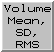### Volume Mean, SD, RMSVolume Mean, SD, RMS calculates statistics for the current set of data in Volume Viewer.

There are several ways to call Volume Mean, SD, RMS, a tool in the Volume Data category (including from the Volume Viewer Tools menu). It is also implemented as the command measure mapStats. For normalizing the data, see vop scale.

Calling the tool calculates the mean, the standard deviation (SD) from the mean, and the root-mean-square (RMS) deviation from zero for the current set of data and reports the results in the Reply Log. Only data for the current subregion and step size are used. SD and RMS are both root-mean-square deviations, but the SD is calculated relative to the average value (mean) whereas the RMS is calculated relative to zero.

UCSF Computer Graphics Laboratory / May 2014